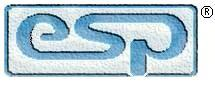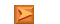Elliott Sound Products Essential Electronic Formulae

# The Formulae You Need To Work With ElectronicsMain IndexArticles Index
Contents
Introduction

There are quite a few formulae (or 'formulas' if you prefer) that are the building blocks of all electronics.  I only intend to cover the basics, so you won't find the formulae for complex filters or anything else out of the ordinary.  This info is covered in more detail in the beginner articles, but here I've concentrated on the basic formulae and nothing else.

Think of this short article as being the 'go-to' place to find the formula you need without much by way of illustration or extensive descriptive text.

In all cases below, resistance is in ohms, capacitance is in Farads and inductance is in Henrys.  If you use megohms and microfarads the result will be the same, but is usually easier to calculate.  If you use a scientific calculator (forget basic pocket calculators as they are useless), a microfarad is entered as 1E-6.  A calculator that uses engineering mode is always better, because it will set all values to multiples of three, so you don't get 'awkward' values like (for example) 1.414E-4  (141.4µ or 141.4E-6).  Common engineering values are as follows ...

 Pico 1e-12 Nano 1e-09 Milli 0.001 Units 1 Kilo 1000 Mega 1e+06 Giga 1e+09 Tera 1e+12

The last two aren't common for most circuitry, but you may come across them in a few applications.

Numerators & Denominators

These two terms often confuse people not used to working with maths.  The numerator is the number at the top of an equation, and can be thought of as describing the number of parts described in the denominator (which is at the bottom).  For example, the fraction 1/4 means that you have one of the four 'parts' - one quarter.  The reciprocal is the decimal rendering of the fraction, in this case 0.25 or 250m (milli).  Not all formulae describe fractions - especially those in electronics, where the goal is to find the decimal value.  No-one wants to deal with 1/1,000,000 Farad capacitors - that's simply 1µF.

The fraction X/Y means 'X pieces of a whole object that is divided into Y equally sized parts'.

1 - Ohm's Law

The most fundamental of all.  R is resistance in ohms, V is voltage and I is current.

R = V / I
V = R × I
I = V / R

Hint: if R is in kΩ then the answer is in milliamps.  1V across 1k gives 1mA.

The total resistance with resistors in series is simply the sum of the resistors.  3 x 1k resistors in series is 3k.  Parallel resistors are a bit trickier.  However, if they're the same value it's easy - 3 × 1k resistors in parallel gives 1/3k, or 333.33Ω.

R = ( R1 × R2 ) / ( R1 + R2 ) ... or ...
R = 1 / (( 1 / R1 ) + ( 1 / R2 ) + ( 1 / Rn ))     (Rn is the nth parallel resistor)

Most calculators provide the reciprocal (1/X), and this makes the second equation much easier to use, and it works with multiple resistors.  The first formula falls apart with three or more variables.  Remember to include the outer set of brackets (ellipses) in the denominator - the bottom part of the equation.

2 - Capacitive Reactance

You don't need it often, but determining capacitive reactance is fundamental to some circuits.  Xc is reactance (impedance) in ohms, C is capacitance in Farads and f is frequency in Hz.  Pi (π) is the standard constant of 3.141592654 (3.141 is close enough, and it's available from nearly all calculators).

Xc = 1 / ( 2π × C × f )
C = 1 / ( 2π × Xc × f )
f = 1 / ( 2π × Xc × C )

The total capacitance with caps in parallel is simply the sum of the capacitors.  3 x 10µF caps in parallel is 30µF.  This time, series caps are a bit trickier.

C = ( C1 × C2 ) / ( C1 + C2 ) ... or ...
R = 1 / (( 1 / C1 ) + ( 1 / CR2 ) + ( 1 / Cn ))     (Cn is the nth parallel capacitor)

The same comments apply as shown for resistors.

3 - Inductive Reactance

More common than capacitive reactance, and inductive reactance is often needed when coils are used.  XL is reactance (impedance) in ohms, L is inductance in Henrys and f is frequency in Hz.

XL = 2π × L × f
L = XL / ( 2π × f )
f = XL / ( 2π × L )

The total inductance with coils in series is simply the sum of the inductors.  3 x 1H inductors in series is 3H.  Parallel inductors are determined in the same way as resistors.

L = ( L1 × L2 ) / ( L1 + L2 ) ... or ...
L = 1 / (( 1 / L1 ) + ( 1 / L2 ) + ( 1 / Ln ))     (Ln is the nth parallel inductor)

4 - Resonance & Filters

Basic resistor/ capacitor (R/C) filters can be high or low pass.  A high-pass filter is also called a differentiator, and a low-pass filter is an integrator.  Only single pole (1st order or 6dB/ octave) networks are described here, and the formula is the same for high and low pass filters.  Whether it is high or low pass depends on the way the two components are wired.

f = 1 / ( 2π × R × C )
R = 1 / ( 2π × f × C )
C = 1 / ( 2π × f × R )

'f' is the -3dB frequency, and the output voltage is 0.707 (1/√2) times the input voltage.  With 1V input, there is 0.707V across the resistor and capacitor, and the output phase is shifted by 90° with respect to the input.

R/C networks also have a time constant, which is usually only needed for timing circuits.  Note that some filters may be described in terms of time constant rather than -3dB frequency (for example the RIAA vinyl disc replay EQ curve).

t = R × C
R = t / C
C = t / R
f = 1 / ( 2π × t )

Inductor/ capacitor (L/C) filters are far more complex, and I will only provide the formulae for resonance.  Q (quality factor), bandwidth and other parameters are not covered.  L/C filters can be in series or parallel, but if we ignore the inductor's series resistance the formula is the same for both types.

f = 1 / ( 2π × √( L × C ))
L = 1 / ( 4 × π ² × f ² × C )
C = 1 / ( 4 × π ² × f ² × L )

The impedance of a resonant filter depends on the topology.  Theoretically 'ideal' series resonant filters have zero impedance at resonance, and parallel resonant circuits have an infinite impedance at resonance.  All real-world filters will have series resistance which changes the behaviour, but only slightly in a well designed circuit with optimised components.

7 - Sound Pressure Level (SPL)
 Continuous dB SPL Maximum Exposure Time 85 8 hours 88 4 hours 91 2 hours 94 1 hour 97 30 minutes 100 15 minutes 103 7.5 minutes 106 < 4 minutes 109 < 2minutes 112 ~ 1 minute 115 ~ 30 seconds
Table 1 - Maximum Exposure to SPLMain IndexArticles Index
 Copyright Notice. This article, including but not limited to all text and diagrams, is the intellectual property of Rod Elliott, and is © 2015.  Reproduction or re-publication by any means whatsoever, whether electronic, mechanical or electro- mechanical, is strictly prohibited under International Copyright laws.  The author (Rod Elliott) grants the reader the right to use this information for personal use only, and further allows that one (1) copy may be made for reference.  Commercial use is prohibited without express written authorisation from Rod Elliott.